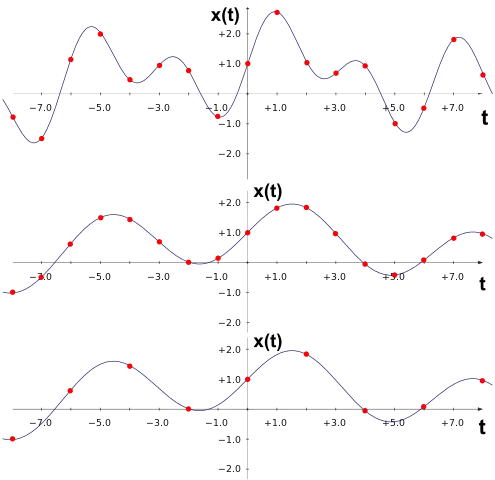# Mathematics - Signals And Systems - Discrete-Time Sampling[Image1]

## Introduction

Hey it's a me again @drifter1!

Today we continue with my mathematics series about Signals and Systems in order to cover Discrete-Time Sampling.

So, without further ado, let's dive straight into it!

## Getting into Discrete-Time Sampling

Until now, we used sampling as a way of processing continuous-time signals using discrete-time systems. But, sampling can also be applied to discrete-time signals, which in turn gives us the concept of discrete-time sampling (or Frequency Domain Sampling). The procedure is also quite similar to the one we used for continuous-time signals, in the sense that the discrete-time sequence is again multiplied by an periodic impulse train. With a period of N, this impulse train, might be used to retain every Nth sample of the original sequence, whilst the rest stays at zero. Of course, the same constraints of bandwidth also apply for discrete-time sampling, and so the original sequence can be recovered under the constraints of the sampling theorem. Furthermore, interpolation can again be implemented using an ideal low-pass filter.

## Downsampling (or Decimation)

We can easily conclude that discrete-time sampling is quite similar to continuous-time sampling, so where is the difference? Well, discrete-time sampling is more of a downsampling or decimation procedure. After sampling the sequence, the newly obtained sequence has no practical reason to retain the zero values. Its explicitly used for retaining only every Nth sample, whilst sampling continuous-time signals is more of a way to approximate it.

Let's think about the special case where a continuous-time signal is sampled twice, in two steps. The second sampling that happens on the discrete-time representation (in the frequency domain) of course has to use a lower sampling rate then the one that was used originally. This basically means that sampling directly using this lower sampling rate would give the same signal, but in one step.

As we already mentioned in previous parts of the series, the sampling rate can have a quite important effect on the outcome of sampling. If the signal is periodic, a lower sampling rate (and so a decimation or downsampling) might still allow reconstruction, but in other cases it might be impossible to recover the original signal:[Image 2]

## Upsampling

The attempt of reconstructing the original sequence can be refered to as upsampling. This process can be split into 2 stages:
1. The N - 1 zero values that were removed while sampling are re-inserted between the sample points.
2. Interpolation is applied with a low-pass filter to re-construct the original sequence.[Image 3]

Downsampling and upsampling can be used in lots of practical applications. As we already saw previously, one of them is sampling rate conversion. Incompatible systems can be made compatible by introducing such a sampling conversion layer.

## RESOURCES:

### Images

1.2.3.Block diagrams and other visualizations were made using draw.io and GeoGebra

## Final words | Next up

And this is actually it for today's post!

From next time we will start getting into full-on examples/exercises on Filtering, Sampling, Modulation, Interpolation etc.

See Ya!Keep on drifting!

H2
H3
H4
3 columns
2 columns
1 column